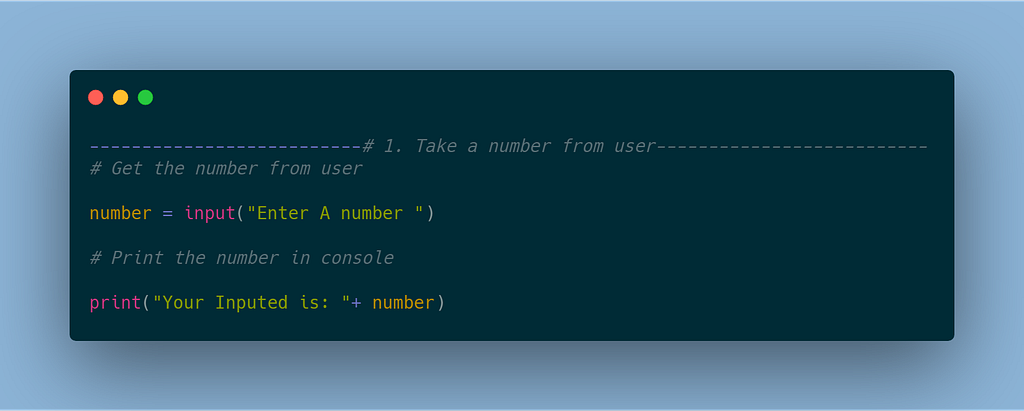How to Solve a Programming Problem

23rd May 2019 Off By binaryCoding without problems is unheard of. Pro coders face a lot of problems when coding. They use Google A LOT. In this article, we’ll try to solve a problem together.

Suppose we have a problem where a user will give the range of numbers and we need to find all the even and print them into the UI or console window.

There are a lot of different ways to follow to solve a problem. You can go with your instinct or you can build a strategy to solve the problem. Let’s find some way together to solve this one together.

Make It Modular

The first option is where we break a problem into smaller portions. We can break our problem into the following:

1. Take a number from the user

2. Find out if it is even or odd

3. Take the range input from the user

4. Loop the whole even or odd process to find the result

5. Finally, print the output value

6. Review Bugs

7. Review the code

8. Optimized the final one

For all coding, we need to do the last 3 steps. All others can be changed as per the problem requirements. We are going to use python for the code.

1. Take a number from the user

We can break this into modules also. First, we take an input from the user then print in the console. We will use the input to get the number from the user.code for get input from the user and print it on console

2. Find out if the number is even or odd

In this part we will think about how we can find out a number is even. If a number has a remainder of 0 after dividing by 2, then the number is even, so the other numbers will be odd. Firstly, we will write some code where we will check if the number is even or odd and we will print that in the console. Here we have two conditions, so we should think of using an if else or switch case. Depends on which one you prefer — just go with it. We will go with if else this time.

3. Take the range input from the user

Now we need to find the even and odd numbers, but in our requirements, we need to take some more data from the user, so let’s do that. In the first input, we will take the first number, and the last number will be taken using another input.

4. Loop the whole even or odd process to find the result

We need a loop now so we can find all the odd and even numbers from the range. We can use many types of loops here, but I like for so I am going with for. You can use whatever you like. We print the even and odd both.

5. Print the output value

Our program is complete, so what do we do now? We need to print the desired output of the user. The user wants us to print even values, so let’s do that. We just have to change it and in else we can use continue to ignore that.

6. Review Bugs

Bug reviews are really important because a bug can mess up the results badly and it can cause a lot of issues. Tester/SQA should not find bugs in your code. It’s your responsibility to make your code bug-free and awesome! You can test using different types of inputs.

7. Review the code

Reviewing code is very important. Everyone should write neat and clean code. Commenting properly is very important, it helps other developers to understand the code easily — so keep commenting more in codes. At a pro level, undocumented code is really hard to learn from.

8. Optimize

Everyone should optimize their code, there are a lot of ways to optimized code, but you do not need not know all of them.